## Tal Mor, Technion-Israel Institute of Technology "From Quantum Computers to Identification of Molecules" Tuesday, August 2, 2005

###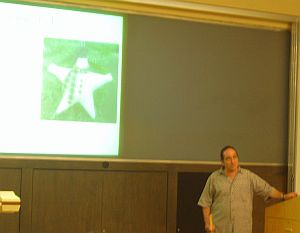Abstract:
In this talk I will suggest the first near-future application of quantum computing devices, “Algorithmic Cooling”. I will explain how simple quantum algorithms, and novel entropy manipulations (that go far beyond Shannon’s entropy bound), can be combined in order to improve identification of molecules. Molecules are built from atoms, and the nucleus inside each atom has a property called spin. The spin can be understood as the orientation of the nucleus, and when put in a magnetic field, certain spins are binary, either up (ZERO) or down (ONE). Several such bits (inside one molecule) represent a binary string -- a register. A macroscopic number of such registers/molecules are monitored in parallel, as done, for instance, in Magnetic Resonance Imaging (MRI). The device that monitors and manipulates these bits/spins is considered a simple “computing” device. Our goal is to improve the molecules’ identification process by using the computing device to run “data compression” algorithms that reduce the entropy of certain spins. A bit with lower entropy is considered “cooler”, and it provides a better signal when used for identifying molecules. Shannon's entropy bound tells us that the total entropy of the spins in a molecule is preserved. Therefore, cooling one spin is done at the expense of heating the others. Our “Algorithmic Cooling” employs data compression methods in *open systems*, thus reducing the entropy of spins far beyond Shannon's bound. Cooling of short molecules is experimentally feasible -- we recently cooled spins of a three-bit quantum computer beyond Shannon's entropy bound at the Technion NMR lab.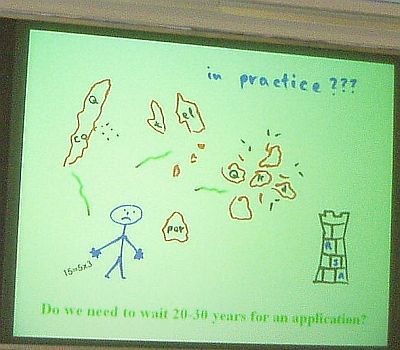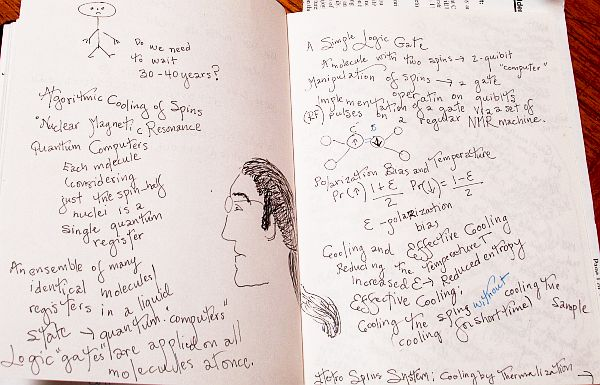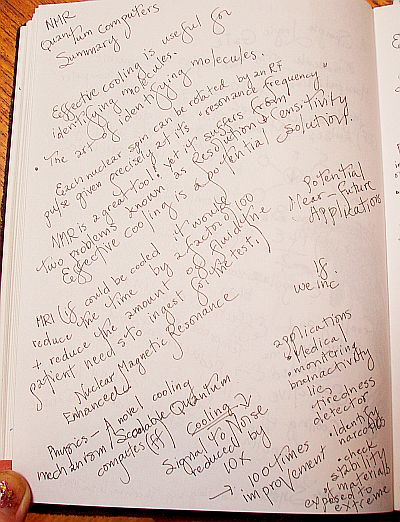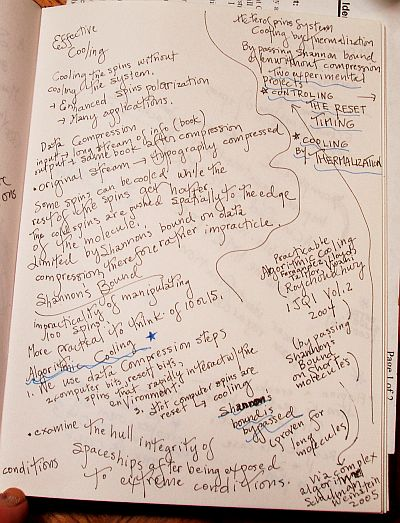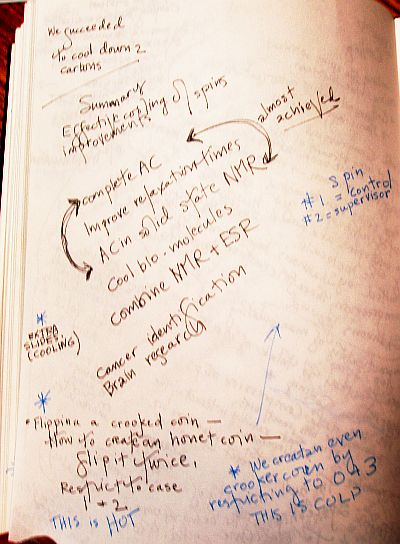For other "Outsiders"
See definitions regarding Algorithms
Regarding "Cooling":
"In genetic programming, this approach is extended to algorithms, by regarding the algorithm itself as a 'solution' to a problem. Also there are heuristic algorithms, whose general purpose is not to find a final solution, but an approximate solution where the time or resources to find a perfect solution are not practical. An example of this would be simulated annealing algorithms, a class of heuristic probabilistic algorithms that vary the solution of a problem by a random amount. The name 'simulated annealing' alludes to the metallurgic term meaning the heating and COOLING of metal to achieve freedom from defects. The purpose of the random variance is to find close to globally optimal solutions rather than simply locally optimal ones, the idea being that the random element will be decreased as the algorithm settles down to a solution."

Go back to the Main page